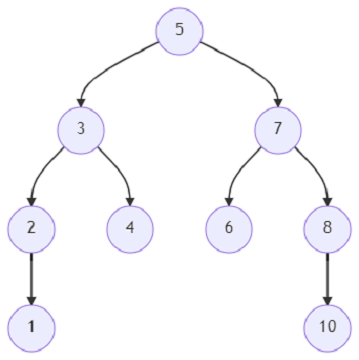# Program to find out the lowest common ancestor of a binary tree using Python

Suppose, we are given a binary tree and also two specific nodes x and y. We have to find out the lowest common ancestor of the two nodes from the binary tree. The lowest common ancestor in a binary tree is the lowest node of which both the nodes x and y are descendants of. Also, a particular node can also be a descendant of itself. We have to find the node and return it as an output.

So, if the input is likeand x = 2, y = 4; then the output will be 3.

The node of which the nodes 2 and 4 are descendant of is 3. So, 3 will be returned.

To solve this, we will follow these steps −

• Define a function dfs() . This will take node

• if node is similar to null, then

• return

• if node is present in list [x,y], then

• left := dfs(left of node)

• right := dfs(right of node)

• if left or right is non-zero, then

• ans := node

• return node

• left := dfs(left of node)

• right := dfs(right of node)

• if left and right is not null, then

• ans := node

• return node

• return left or right

• ans := dfs(root)

• return ans

## Example

Live Demo

class TreeNode:
def __init__(self, data, left = None, right = None):
self.data = data
self.left = left
self.right = right
def insert(temp,data):
que = []
que.append(temp)
while (len(que)):
temp = que
que.pop(0)
if (not temp.left):
if data is not None:
temp.left = TreeNode(data)
else:
temp.left = TreeNode(0)
break
else:
que.append(temp.left)
if (not temp.right):
if data is not None:
temp.right = TreeNode(data)
else:
temp.right = TreeNode(0)
break
else:
que.append(temp.right)
def make_tree(elements):
Tree = TreeNode(elements)
for element in elements[1:]:
insert(Tree, element)
return Tree
def search_node(root, element):
if (root == None):
return None
if (root.data == element):
return root
res1 = search_node(root.left, element)
if res1:
return res1
res2 = search_node(root.right, element)
return res2
def solve(root, x, y):
def dfs(node):
if not node:
return
if node in [x,y]:
left = dfs(node.left)
right = dfs(node.right)
if left or right:
ans = node
return node
left = dfs(node.left)
right = dfs(node.right)
if left and right:
ans = node
return node
return left or right
ans = dfs(root)
return ans
root = make_tree([5, 3, 7, 2, 4, 1, 7, 6, 8, 10])
print(solve(root, search_node(root, 2), search_node(root, 4)).data)

## Input

make_tree([5, 3, 7, 2, 4, 1, 7, 6, 8, 10]),
search_node(root, 2),
search_node(root, 4)

## Output

3